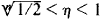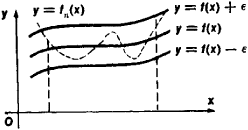# Uniform Convergence

(redirected from Uniformly convergent)

## uniform convergence

[′yü·nə‚fȯrm kən′vər·jəns]
(mathematics)
A sequence of functions {ƒn (x)} converges uniformly on E to ƒ(x) if given ε > 0 there is an N such that |ƒn (x) - ƒ(x)| < ε="" for="" all="">x in E provided n > N.

## Uniform Convergence

an important special case of convergence. A sequence of functions fn (x) (n = 1, 2, 3,…) is said to converge uniformly on a given set to the limit function f(x) if, for every ∊ > 0, there exists a number N = N(∊) such that, when η > N, ǀ f(x) – fn (x)ǀ < ∊ for all points x in the set.

For example, the sequence of functions fn(x) = xn converges uniformly on the closed interval [0, 1 /2] to the limit f(x) = 0. To show that this is true, let us take n > In (1/∊)/In 2. It then follows that ǀf(x)–fn (x)ǀ ≤ (1/2)n < ∊ for all x, 0 ≤ x ≤ 1/2. This sequence of functions, however, does not converge uniformly on the interval [0, 1]. Here, the limit function is f(x) = 0 for 0 ≤ x< 1 and f(1) = 1. The reason for the failure to converge uniformly is that for arbitarily large η there exist points η that satisfy the inequalitiesand for which ǀf (η) - fn(η)ǀ = ηn > 1/2.

The notion of uniform convergence admits of a simple geometric interpretation. The uniform convergence of a sequence of functions fn(x) on some closed interval to the function f(x) means that, for any ∊ > 0, all curves y = fn (x) with large enough n will be located within a strip that is 2∊ in width and is bounded by the curves y = f(x) ± ∊ for any x in the interval (see Figure 1).Figure 1

Uniformly converging sequences of functions have a number of important properties. For example, the limit of a uniformly converging sequence of continuous functions is also continuous. On the other hand, the example given above shows that the limit of a sequence of functions that does not converge uniformly may be discontinuous. An important role is played in mathematical analysis by Weierstrass’ theorem, which states that every function continuous on a closed interval can be represented as the limit of a uniformly converging sequence of polynomials. (SeeAPPROXIMATION AND INTERPOLATION OF FUNCTIONS.)

References in periodicals archive ?
0](0, T) and, as a consequence, it is uniformly convergent in [0, T].
Consequently, it is uniformly convergent in [0, T].
The mapping is derived in terms of the uniformly convergent Poincare series (4.
1) and term-by-term integration of the obtained uniformly convergent series yields the exact formula, (4.
n]) has a subsequence [MATHEMATICAL EXPRESSION NOT REPRODUCIBLE IN ASCII] is uniformly convergent on X for all k (0 [less than or equal to] k [less than or equal to] m).
n]) has a uniformly convergent subsequence [MATHEMATICAL EXPRESSION NOT REPRODUCIBLE IN ASCII] in C(X).
We have seen that for the Hilbert transform the approximation process does not converge uniformly on R, even if the original sampling series is uniformly convergent on R.
It is easy to see that the power series in (3) is absolutely and uniformly convergent in any compact domain in C \ {1/2, -1/2, -3/2, -5/2.
Mean square convergent and uniformly convergent expansions in the eigenfunctions are established.
and so the equations can be solved iteratively to give a uniformly convergent series of "generalized rays" for given [[xi].
pi]] a reconstruction in the form of a sampling series that is uniformly convergent on compact subsets of the real axis and uniformly bounded on the whole real axis is not possible in general if the signals are sampled equidistantly at Nyquist rate.
n]: X [right arrow] X be a sequence of continuous maps uniformly convergent to a function f .

Site: Follow: Share:
Open / Close